• 文章目录平面参数方程平面向量式方程平面的行列方程平面的三点式方程平面的点法式方程平面的一般方程平面的一般方程讨论小结：平面方程的几种形式参考资料 平面是随处可见的空间形状 问题1：如何从几何上确定...


文章目录
平面的参数方程平面的向量式方程平面的行列式方程平面的三点式方程
平面的点法式方程平面的一般方程平面的一般方程讨论
小结：平面方程的几种形式参考资料

06向量及其坐标表示、向量的方向角与方向余弦、向量组共线与共面的条件、向量的加法与数乘运算、向量组的线性组合、二维向量的基向量分解、三维向量的基向量分解、用坐标做向量的数乘07向量的点积、数量积、两向量垂直的条件、投影与投影向量、向量的正交分解、几个不等式、用坐标计算数量积08向量的叉积、向量积、用坐标行列式计算向量积、二重外积09向量的混合积、向量之间的位置关系、用坐标行列式计算混合积、三向量共面的条件10空间直线方程、参数方程、向量式方程、点向式方程、两点式方程、一般方程、空间直线的一般方程化为点向式方程11空间平面方程、参数方程、向量式方程、行列式方程、三点式方程、点法式方程、一般方程
平面是随处可见的空间形状问题1：如何从几何上确定一个平面？不在一条直线上的三点确定一个平面 ．过一定点且垂直于一定直线可以作一个平面．一条直线和直线外一点确定一个平面．两相交直线确定一个平面．两平行直线确定一个平面．
问题2：如何从代数上描述一个平面 ？
用代数方程式刻画平面上动点的轨迹，即建立平面的方程 .
在直角坐标系下，建立动点坐标满足的方程式 .
平面的参数方程
不在一条直线上的三点确定一个平面
要素：点

M

0

(

x

0

,

y

0

,

z

0

)

,

M_{0}\left(x_{0}, y_{0}, z_{0}\right),

不共线的向量

a

,

b

\boldsymbol{a}, \boldsymbol{b}

（已知要素）
任务：求平面

π

\pi

的方程即动点

𝑀

(

𝑥

,

𝑦

,

𝑧

)

𝑀(𝑥, 𝑦, 𝑧)

的轨迹方程．
依据：

M

0

M

→

\overrightarrow{M_{0} M}

与不共线的向量 𝒂 , 𝒃 共面 .关系式：

M

0

M

→

=

λ

a

+

μ

b

\large{\color{red}{\overrightarrow{M_{0} M}=\lambda \boldsymbol{a}+\mu \boldsymbol{b}}}

a

=

(

a

1

,

a

2

,

a

3

)

,

b

=

(

b

1

,

b

2

,

b

3

)

\boldsymbol{a}=\left(a_{1}, a_{2}, a_{3}\right),\boldsymbol{b}=\left(b_{1}, b_{2}, b_{3}\right)

得到：

π

:

{

x

=

x

0

+

λ

a

1

+

μ

b

1

y

=

y

0

+

λ

a

2

+

μ

b

2

z

=

z

0

+

λ

a

3

+

μ

b

3

(1)

\boldsymbol{\pi}:\left\{\begin{array}{l}x=x_{0}+\lambda a_{1}+\mu b_{1} \\ y=y_{0}+\lambda a_{2}+\mu b_{2} \\ z=z_{0}+\lambda a_{3}+\mu b_{3}\end{array}\right.\tag1

称为平面

π

\pi

的参数方程

.

.

其中

λ

,

μ

\lambda, \mu

为参数。
平面上的点都满足方程 。满足方程的点都在平面上， 不在平面上的点不满足方程 .
平面的向量式方程M

0

M

→

=

λ

a

+

μ

b

\large{\color{red}{\overrightarrow{M_{0} M}=\lambda \boldsymbol{a}+\mu \boldsymbol{b}}}

，由

r

=

O

M

→

=

(

x

,

y

,

z

)

r

0

=

O

M

0

→

=

(

x

0

,

y

0

,

z

0

)

r=\overrightarrow{O M}=(x,y,z)\\ r_0=\overrightarrow{O M_0}=(x_0,y_0,z_0)

得到：

π

:

r

=

r

0

+

λ

a

+

μ

b

.
称为平面的向量式方程.
平面的行列式方程
要素：点

M

0

(

x

0

,

y

0

,

z

0

)

,

M_{0}\left(x_{0}, y_{0}, z_{0}\right),

不共线的向量

a

,

b

\boldsymbol{a}, \boldsymbol{b}

（已知要素）
依据：三向量

M

0

M

→

,

a

,

b

共面,则它们的混合积为 0 .
平面

π

\pi

的行列式方程为

∣

x

−

x

0

y

−

y

0

z

−

z

0

a

1

a

2

a

3

b

1

b

2

b

3

∣

=

0

\left|\begin{array}{ccc} x-x_{0} & y-y_{0} & z-z_{0} \\ a_{1} & a_{2} & a_{3} \\ b_{1} & b_{2} & b_{3} \end{array}\right|=0

平面的三点式方程
要素： 不在一条直线上的三点

M

1

(

x

1

,

y

1

,

z

1

)

,

M

2

(

x

2

,

y

2

,

z

2

)

,

M

3

(

x

3

,

y

3

,

z

3

)

M_{1}\left(x_{1}, y_{1}, z_{1}\right), M_{2}\left(x_{2}, y_{2}, z_{2}\right), M_{3}\left(x_{3}, y_{3}, z_{3}\right)

依据：三向量

M

1

M

→

,

M

1

M

2

→

,

M

1

M

3

→

共面

.

\text { 依据：三向量 } \overrightarrow{M_{1} M}, \overrightarrow{M_{1} M_{2}}, \overrightarrow{M_{1} M_{3}} \text { 共面 } .平面

π

的方程为

\text { 平面 } \pi \text { 的方程为 }

∣

x

−

x

1

y

−

y

1

z

−

z

1

x

2

−

x

1

y

2

−

y

1

z

2

−

z

1

x

3

−

x

1

y

3

−

y

1

z

3

−

z

1

∣

=

0

\begin{array}{l} \left|\begin{array}{ccc} x-x_{1} & y-y_{1} & z-z_{1} \\ x_{2}-x_{1} & y_{2}-y_{1} & z_{2}-z_{1} \\ x_{3}-x_{1} & y_{3}-y_{1} & z_{3}-z_{1} \end{array}\right|=0 \end{array}

一称为平面

π

\pi

的三点式方程
平面的点法式方程
过一定点且垂直于一定直线可以作一个平面．
平行于定直线的非零向量是垂直于平面的，称为平面的法向量.
要素：点

M

0

(

x

0

,

y

0

,

z

0

)

,

M_{0}\left(x_{0}, y_{0}, z_{0}\right),

法向量

n

n

。
任何垂直于平面的非零向量

n

n

都是平面的法向量．与

n

n

平行的所有非零向量均可作为此平面的法向量．平面上的所有向量都与该平面的法向量垂直．要素：点

M

0

(

x

0

,

y

0

,

z

0

)

,

M_{0}\left(x_{0}, y_{0}, z_{0}\right),

法向量

n

=

(

A

,

B

,

C

)

\boldsymbol{n}=(A, B, C)

.
设

M

(

x

,

y

,

z

)

M(x, y, z)

为平面上的动点, 则

n

⊥

M

0

M

→

,

向

量

关

系

\boldsymbol{n} \perp \overrightarrow{M_{0} {M}} ,~~~~~~ 向量关系

有

n

⋅

M

0

M

→

=

0.

向量代数关系

\begin{array}{lll}\text { 有 } & \boldsymbol{n} \cdot \overrightarrow{\boldsymbol{M}_{0} \boldsymbol{M}}=\mathbf{0} . & \text { 向量代数关系 }\end{array}

平面

π

\pi

的点法式方程

.

.

:

π

:

A

(

x

−

x

0

)

+

B

(

y

−

y

0

)

+

C

(

z

−

z

0

)

=

0

\pi: A\left(x-x_{0}\right)+B\left(y-y_{0}\right)+C\left(z-z_{0}\right)=0

平面的一般方程
点法式 ：

π

:

A

(

x

−

x

0

)

+

B

(

y

−

y

0

)

+

C

(

z

−

z

0

)

=

0

\pi: A\left(x-x_{0}\right)+B\left(y-y_{0}\right)+C\left(z-z_{0}\right)=0

,转化得到

π

:

A

x

+

B

y

+

C

z

+

D

=

0

\pi: A x+B y+C z+D=0

称为平面

π

\pi

的一般方程,其中

n

=

(

A

,

B

,

C

)

n=(A, B, C)

为平面的法向量
【注】 平面方程是一个三元一次方程 .反之，一个三元一次方程在几何上表示一个平面 .
平面的一般方程讨论

π

:

A

x

+

B

y

+

C

z

+

D

=

0

(

A

,

B

,

C

\pi: A x+B y+C z+D=0 \quad(A, B, C

不全为0

)

)

(1) 当

D

=

0

D=0

时, 平面

π

:

A

x

+

B

y

+

C

z

=

0

\pi: A x+B y+C z=0

过原点;
(2) 当

A

=

0

A=0

时, 平面

π

:

B

y

+

C

z

+

D

=

0

\pi: B y+C z+D=0

平行于

x

x

轴;
当

B

=

0

B=0

时, 平面

π

:

A

x

+

C

z

+

D

=

0

\pi: A x+C z+D=0

平行于

y

y

轴
当

C

=

0

C=0

时, 平面

π

:

A

x

+

B

y

+

D

=

0

\pi: A x+B y+D=0

平行于

z

z

轴
(3) 当

A

=

0

,

D

=

0

A=0, D=0

时, 平面

π

:

B

y

+

C

z

=

0

\pi: B y+C z=0

过

x

x

轴;
当

B

=

0

,

D

=

0

B=0, D=0

时, 平面

π

:

A

x

+

C

z

=

0

\pi: A x+C z=0

过

y

y

轴;
当

C

=

0

,

D

=

0

C=0, D=0

时, 平面

π

:

A

x

+

B

y

=

0

\pi: A x+B y=0

过

z

z

轴
(4) 当

A

=

0

,

B

=

0

A=0, B=0

时, 平面

π

:

C

z

+

D

=

0

\pi: C z+D=0

平行于

x

O

y

x O y

面
当

B

=

0

,

C

=

0

B=0, C=0

时, 平面

π

:

A

x

+

D

=

0

\pi: A x+D=0

平行于

y

O

z

y O z

面
当

A

=

0

,

C

=

0

A=0, C=0

时，平面

π

:

B

y

+

D

=

0

\pi: B y+D=0

平行于

z

O

x

z O x

面.

例

1

\Large\color{violet}{例1}

求过

M

0

(

2

,

1

,

1

)

M_{0}(2,1,1)

且平行于

π

1

:

x

+

2

y

−

3

z

+

7

=

0

\pi_{1}: x+2 y-3 z+7=0

的平面方程
【解法一】 因所求平面

π

\pi

与已知平面

π

1

\pi_{1}

平行, 则

π

1

\pi_{1}

的法向量

n

=

(

1

,

2

,

−

3

)

n=(1,2,-3)

也是

π

\pi

的法向量，则平面

π

\pi

的点法式方程为

1

⋅

(

x

−

2

)

+

2

⋅

(

y

−

1

)

+

(

−

3

)

⋅

(

z

−

1

)

=

0

1 \cdot(x-2)+2 \cdot(y-1)+(-3) \cdot(z-1)=0

整理得平面

π

\pi

的一般方程为

x

+

2

y

−

3

z

−

1

=

0

x+2 y-3 z-1=0

【解法二 】因所求平面

π

\pi

与已知平面

π

1

\pi_{1}

平行, 设平面

π

\pi

的一般方程为

x

+

2

y

−

3

z

+

D

=

0

x+2 y-3 z+D=0

代入点

M

0

(

2

,

1

,

1

)

M_{0}(2,1,1)

的坐标, 得

2

+

2

−

3

+

D

=

0

,

故

D

=

−

1.

D=-1 .

于是平面

π

\pi

的方程为

x

+

2

y

−

3

z

−

1

=

0

x+2 y-3 z-1=0

例

2

\Large\color{violet}{例2}

已知一平面

π

\pi

与三个坐标轴的交点分别为

P

(

a

,

0

,

0

)

,

Q

(

0

,

b

,

0

)

,

R

(

0

,

0

,

c

)

(

a

,

b

,

c

≠

0

)

求平面

π

\pi

的方程 .
【解法一】由平面的三点式方程知

∣

x

−

a

y

−

0

z

−

0

0

−

a

b

−

0

0

−

0

0

−

a

0

−

0

c

−

0

∣

=

0

\left|\begin{array}{ccc} x-a & y-0 & z-0 \\ 0-a & b-0 & 0-0 \\ 0-a & 0-0 & c-0 \end{array}\right|=0

整理得平面

π

\pi

的一般方程为

b

c

x

+

a

c

y

+

a

b

z

−

a

b

c

=

0.

\quad b c x+a c y+a b z-a b c=0 .

进一步整理得

π

:

x

a

+

y

b

+

z

c

=

1.

⟶

称为平面

π

\pi

的

截

距

式

方

程

\large\color{#70f3ff}{\boxed{\color{green}{截距式方程}}}

【解法二 】 设平面

π

\pi

的一般方程为

π

:

A

x

+

B

y

+

C

z

+

D

=

0

,

\pi: A x+B y+C z+D=0,

则

{

A

a

+

D

=

0

B

b

+

D

=

0

C

c

+

D

=

0

\left\{\begin{array}{l} A a+D=0 \\ B b+D=0 \\ C c+D=0 \end{array}\right.

解得

A

=

−

D

a

,

B

=

−

D

b

,

C

=

−

D

c

.

A=-\frac{D}{a}, B=-\frac{D}{b}, C=-\frac{D}{c} .

因

A

,

B

,

C

A, B, C

不全为

0

,

0,

则

D

≠

0

D \neq 0

. 于是得

π

:

x

a

+

y

b

+

z

c

=

1

例

3

\Large\color{violet}{例3}

已知空间四点

A

(

1

,

0

,

0

)

,

B

(

2

,

1

,

3

)

,

C

(

1

,

2

,

5

)

,

P

(

2

,

3

,

t

)

A(1,0,0), B(2,1,3), C(1,2,5), P(2,3, t)

问：当

t

t

为何值时, 这四点在一个平面上? 并求出该平面方程.
【解 】

A

(

1

,

0

,

0

)

,

B

(

2

,

1

,

3

)

,

C

(

1

,

2

,

5

)

,

P

(

2

,

3

,

t

)

A(1,0,0), B(2,1,3), C(1,2,5), P(2,3, t)

四点共面的充要条件是三向量

A

P

→

=

(

1

,

3

,

t

)

,

A

B

→

=

(

1

,

1

,

3

)

,

A

C

→

=

(

0

,

2

,

5

)

\overrightarrow{A P}=(1,3, t), \overrightarrow{A B}=(1,1,3), \overrightarrow{A C}=(0,2,5)

共面,

故

∣

1

3

t

1

1

3

0

2

5

∣

=

2

t

−

16

=

0

,

即

t

=

8

\left|\begin{array}{lll} 1 & 3 & t \\ 1 & 1 & 3 \\ 0 & 2 & 5 \end{array}\right|=2 t-16=0, \text { 即 } t=8

所以, 当

t

=

8

t=8

时,

A

、

B

、

C

、

P

A 、 B 、 C 、 P

四点共面.
该平面的三点式方程为

∣

x

−

1

y

−

0

z

−

0

2

−

1

1

−

0

3

−

0

1

−

1

2

−

0

5

−

0

∣

=

∣

x

−

1

y

z

1

1

3

0

2

5

∣

=

0

\left|\begin{array}{ccc} x-1 & y-0 & z-0 \\ 2-1 & 1-0 & 3-0 \\ 1-1 & 2-0 & 5-0 \end{array}\right|=\left|\begin{array}{ccc} x-1 & y & z \\ 1 & 1 & 3 \\ 0 & 2 & 5 \end{array}\right|=0

整理得平面的一般方程为

x

+

5

y

−

2

z

−

1

=

0

x+5 y-2 z-1=0

.

注

：

\Large\color{violet}{注：}

也可以先求出由

A

、

B

、

C

A 、 B 、 C

三点所确定的平面的方程

x

+

5

y

−

2

z

−

1

=

0

x+5 y-2 z-1=0

再代入点P的坐标，得

t

=

8

t=8

.
小结：平面方程的几种形式
设点

M

0

(

x

0

,

y

0

,

z

0

)

M_{0}\left(x_{0}, y_{0}, z_{0}\right)

在平面上,

n

=

(

A

,

B

,

C

)

是平面的法向量
1、平面的点法式方程

A

(

x

−

x

0

)

+

B

(

y

−

y

0

)

+

C

(

z

−

z

0

)

=

0

A\left(x-x_{0}\right)+B\left(y-y_{0}\right)+C\left(z-z_{0}\right)=0

2、平面的一般式方程

A

x

+

B

y

+

C

z

+

D

=

0

A x+B y+C z+D=0

3 、平面的截距式方程

x

a

+

y

b

+

z

c

=

1.

其中

a

,

b

,

c

a, b, c

为平面在三个坐标轴上的截距.
4 、平面的三点式方程
设

M

1

(

x

1

,

y

1

,

z

1

)

,

M

2

(

x

2

,

y

2

,

z

2

)

,

M

3

(

x

3

,

y

3

,

z

3

)

M_{1}\left(x_{1}, y_{1}, z_{1}\right), M_{2}\left(x_{2}, y_{2}, z_{2}\right), M_{3}\left(x_{3}, y_{3}, z_{3}\right)

是平面上不共线的三点, 则

∣

x

−

x

1

y

−

y

1

z

−

z

1

x

2

−

x

1

y

2

−

y

1

z

2

−

z

1

x

3

−

x

1

y

3

−

y

1

z

3

−

z

1

∣

=

0

\left|\begin{array}{ccc} x-x_{1} & y-y_{1} & z-z_{1} \\ x_{2}-x_{1} & y_{2}-y_{1} & z_{2}-z_{1} \\ x_{3}-x_{1} & y_{3}-y_{1} & z_{3}-z_{1} \end{array}\right|=0

5 、平面的参数式方程

π

:

{

x

=

x

0

+

λ

a

1

+

μ

b

1

y

=

y

0

+

λ

a

2

+

μ

b

2

,

z

=

z

0

+

λ

a

3

+

μ

b

3

\quad \pi:\left\{\begin{array}{l}x=x_{0}+\lambda a_{1}+\mu b_{1} \\ y=y_{0}+\lambda a_{2}+\mu b_{2}, \\ z=z_{0}+\lambda a_{3}+\mu b_{3}\end{array}\right.

6 、平面的行列式方程

∣

x

−

x

0

y

−

y

0

z

−

z

0

a

1

a

2

a

3

b

1

b

2

b

3

∣

=

0

\quad\left|\begin{array}{ccc}x-x_{0} & y-y_{0} & z-z_{0} \\ a_{1} & a_{2} & a_{3} \\ b_{1} & b_{2} & b_{3}\end{array}\right|=0

.
7 、平面的向量式方程

π

:

r

=

r

0

+

λ

a

+

μ

b

.
参考资料
空间解析几何_国防科技大学
北京大学数学系几何与代数教研室代数小组，《高等代数》（第四版）
高等代数，林亚南，高等教育出版社
高等代数学习辅导，林亚南，林鹭，杜妮，陈清华，高等教育出版社
高等代数 电子科技大学
高等代数_安阳师范学院
《高等代数》（第五版）
展开全文• 文章目录空间直线的参数方程空间直线的向量式方程直线的点向方程空间直线的两点方程空间直线的一般方程空间直线的一般方程化为点向方程小结：直线方程的几种形式参考资料 直线是随处可见的空间形状 问题:如何...


文章目录
空间直线的参数方程空间直线的向量式方程
直线的点向式方程空间直线的两点式方程
空间直线的一般方程空间直线的一般方程化为点向式方程
小结：直线方程的几种形式参考资料

06向量及其坐标表示、向量的方向角与方向余弦、向量组共线与共面的条件、向量的加法与数乘运算、向量组的线性组合、二维向量的基向量分解、三维向量的基向量分解、用坐标做向量的数乘07向量的点积、数量积、两向量垂直的条件、投影与投影向量、向量的正交分解、几个不等式、用坐标计算数量积08向量的叉积、向量积、用坐标行列式计算向量积、二重外积09向量的混合积、向量之间的位置关系、用坐标行列式计算混合积、三向量共面的条件10空间直线方程、参数方程、向量式方程、点向式方程、两点式方程、一般方程、空间直线的一般方程化为点向式方程11空间平面方程、参数方程、向量式方程、行列式方程、三点式方程、点法式方程、一般方程
直线是随处可见的空间形状问题:如何从几何上确定一个空间直线?
过一定点可以作唯一直线平行于一定直线.两点可以确定一条直线．任意一条直线可以视为两相交平面的交线.空间直线的参数方程
过一定点可以作唯一直线平行于一定直线.
任何平行于直线的非零向量

v

v

称为直线的方向向量．
要素：点

M

0

(

x

0

,

y

0

,

z

0

)

,

M_{0}\left(x_{0}, y_{0}, z_{0}\right),

方向向量

v

=

(

m

,

n

,

p

)

v=(m, n, p)

.
与

v

v

平行的所有非零向量均可作为此直线的方向向量． 直线上的所有向量都与该直线的方向向量平行．
任务：求直线

𝐿

𝐿

的方程即动点

𝑀

(

𝑥

,

𝑦

,

𝑧

)

𝑀(𝑥, 𝑦, 𝑧)

的轨迹方程．
依据：

M

0

M

→

\overrightarrow{M_{0} M}

与方向向量

v

v

平行 .
关系：

M

0

M

→

=

t

v

\large{\color{red}{\overrightarrow{M_{0} M}=t \boldsymbol{v}}}要

素

\Large\color{violet}{要素}

点

M

0

(

x

0

,

y

0

,

z

0

)

,

M_{0}\left(x_{0}, y_{0}, z_{0}\right),

方向向量

v

=

(

m

,

n

,

p

)

v=(m, n, p)

.
关系：

M

0

M

→

=

t

v

\large{\color{red}{\overrightarrow{M_{0} M}=t \boldsymbol{v}}}

,得到

直

线

𝑳

的

参

数

方

程

\large\color{#70f3ff}{\boxed{\color{green}{直线 𝑳 的参数方程}}}

L

:

{

x

=

x

0

+

m

t

y

=

y

0

+

n

t

,

−

∞

<

t

<

+

∞

.

z

=

z

0

+

p

t

【注1】 直线方程只含一个自由参数，通常说直线是一维的 .
【注2】 直线可以看作匀速运动的质点的轨迹，其中 𝒗 是速度，𝑡是时间.
空间直线的向量式方程
关系：

M

0

M

→

=

t

v

\large{\color{red}{\overrightarrow{M_{0} M}=t \boldsymbol{v}}}由

r

=

O

M

→

=

(

x

,

y

,

z

)

r=\overrightarrow{O M}=(x, y, z)

，

r

0

=

O

M

0

→

=

(

x

0

,

y

0

,

z

0

)

r_0=\overrightarrow{O M_0}=(x_0, y_0, z_0)

可以得到

L

=

r

0

+

t

v

⟶

{L} =r_{0}+t v\longrightarrow

直

线

𝑳

的

向

量

式

方

程

\large\color{#70f3ff}{\boxed{\color{green}{直线 𝑳 的向量式方程}}}

直线的点向式方程
要素：点

M

0

(

x

0

,

y

0

,

z

0

)

,

M_{0}\left(x_{0}, y_{0}, z_{0}\right),

方向向量

v

=

(

m

,

n

,

p

)

v=(m, n, p)

.
依据：

M

0

M

→

\overrightarrow{M_{0} M}

与方向向量

v

v

平行

.

.

关系：

M

0

M

→

×

v

=

0

或

M

0

M

→

=

t

v

\large{\color{red}{ \overrightarrow{M_{0} M} \times \boldsymbol{v}=\mathbf{0} \text { 或 } \overrightarrow{M_{0} M}=t \boldsymbol{v}}}

L

:

x

−

x

0

m

=

y

−

y

0

n

=

z

−

z

0

p

.

\begin{array}{l} L: \frac{x-x_{0}}{m}=\frac{y-y_{0}}{n}=\frac{z-z_{0}}{p} . \end{array}

——称为直线

L

L

的

点

向

式

（

标

准

、

对

称

式

）

方

程

\large\color{#70f3ff}{\boxed{\color{green}{点向式（标准、对称式）方程}}}

.
空间直线的两点式方程
两点可以确定一条直线 ：
要素： 点

M

1

(

x

1

,

y

1

,

z

1

)

,

M

2

(

x

2

,

y

2

,

z

2

)

M_{1}\left(x_{1}, y_{1}, z_{1}\right), M_{2}\left(x_{2}, y_{2}, z_{2}\right)

要素： 点

M

1

(

x

0

,

y

0

,

z

0

)

,

M_{1}\left(x_{0}, y_{0}, z_{0}\right),

方向向量

v

=

M

1

M

2

→

\boldsymbol{v}=\overrightarrow{M_{1} M_{2}}

L

:

x

−

x

1

x

2

−

x

1

=

y

−

y

1

y

2

−

y

1

=

z

−

z

1

z

2

−

z

1

L: \frac{x-x_{1}}{x_{2}-x_{1}}=\frac{y-y_{1}}{y_{2}-y_{1}}=\frac{z-z_{1}}{z_{2}-z_{1}}

——称为直线 𝑳 的

两

点

式

方

程

\large\color{#70f3ff}{\boxed{\color{green}{两点式方程}}}

空间直线的一般方程
任意一条直线可以视为两相交平面的交线 ．过直线 𝐿 的两平面 :

π

1

:

A

1

x

+

B

1

y

+

C

1

z

+

D

1

=

0

π

2

:

A

2

x

+

B

2

y

+

C

2

z

+

D

2

=

0

\pi_{1}: A_{1} x+B_{1} y+C_{1} z+D_{1}=0 \\ \pi_{2}: A_{2} x+B_{2} y+C_{2} z+D_{2}=0

得到直线 𝑳

一

般

方

程

\large\color{#70f3ff}{\boxed{\color{green}{一般方程}}}

：

L

:

{

A

1

x

+

B

1

y

+

C

1

z

+

D

1

=

0

A

2

x

+

B

2

y

+

C

2

z

+

D

2

=

0

\begin{array}{r} L:\left\{\begin{array}{l} A_{1} x+B_{1} y+C_{1} z+D_{1}=0 \\ A_{2} x+B_{2} y+C_{2} z+D_{2}=0 \end{array}\right. \end{array}

【

注

1

】

\Large\color{violet}{【注1】}

在空间直线的一般方程中，对应的两平面的法向量

n

1

=

(

A

1

,

B

1

,

C

1

)

,

n

2

=

(

A

2

,

B

2

,

C

2

)

\boldsymbol{n}_{1}=\left(A_{1}, B_{1}, C_{1}\right), \boldsymbol{n}_{2}=\left(A_{2}, B_{2}, C_{2}\right)

是不平行的, 或者说方程组

{

A

1

x

+

B

1

y

+

C

1

z

+

D

1

=

0

A

2

x

+

B

2

y

+

C

2

z

+

D

2

=

0

\left\{\begin{array}{l} A_{1} x+B_{1} y+C_{1} z+D_{1}=0 \\ A_{2} x+B_{2} y+C_{2} z+D_{2}=0 \end{array}\right.

的系数矩阵

A

=

[

A

1

B

1

C

1

A

2

B

2

C

2

]

A=\left[\begin{array}{lll} A_{1} & B_{1} & C_{1} \\ A_{2} & B_{2} & C_{2} \end{array}\right]

的秩

r

(

A

)

=

2

,

\mathrm{r}(A)=2,

通俗地说, 这两个方程是独立的

.

.

空间直线的一般方程化为点向式方程
因直线

L

L

同时位于平面

π

1

\pi_{1}

和

π

2

\pi_{2}

上, 故直线

L

L

同时垂直于这两个平面的法向量. 所以, 直线

L

L

的方向向量可取为

v

=

n

1

×

n

2

=

∣

i

j

k

A

1

B

1

C

1

A

2

B

2

C

2

∣

\boldsymbol{v}=\boldsymbol{n}_{1} \times \boldsymbol{n}_{2}=\left|\begin{array}{ccc} \boldsymbol{i} & \boldsymbol{j} & \boldsymbol{k} \\ A_{1} & B_{1} & C_{1} \\ A_{2} & B_{2} & C_{2} \end{array}\right|

再取

M

0

(

x

0

,

y

0

,

z

0

)

满足方程组则可将直线的一般式方程转化为点向式方程 .

例

1

\Large\color{violet}{例1}

求过点

M

0

(

1

,

2

,

1

)

M_{0}(1,2,1)

且与平面

π

:

2

x

−

y

+

5

=

0

\pi: 2 x-y+5=0

垂直的直线

L

L

的标准方程、参数方程与一般方程，并求直线

L

L

与

π

\pi

平面的交点坐标.
【解】 因

L

⊥

π

,

L \perp \pi,

则

v

/

/

n

=

(

2

,

−

1

,

0

)

v / / n=(2,-1,0)

.
因此, 所求直线

L

L

的标准方程为

x

−

1

2

=

y

−

2

−

1

=

z

−

1

0

.
它的参数方程为

{

x

=

1

+

2

t

y

=

2

−

t

,

z

=

1

\left\{\begin{array}{c}x=1+2 t \\ y=2-t, \\ z=1\end{array} \quad\right.

它的一般方程为

{

x

+

2

y

−

5

=

0

z

=

1

\left\{\begin{array}{c}x+2 y-5=0 \\ z=1\end{array}\right.

将直线L的参数方程代入平面

π

\pi

的一般方程中得

5

t

+

5

=

0

,

5 t+5=0,

故

t

=

−

1.

t=-1 .

因此, 所求交点为

P

(

−

1

,

3

,

1

)

P(-1,3,1)

.

例

2

\Large\color{violet}{例2}

求过点

M

0

(

2

,

4

,

0

)

M_{0}(2,4,0)

且与直线

L

1

:

{

x

+

2

z

−

1

=

0

,

y

−

3

z

−

2

=

0

L_{1}:\left\{\begin{array}{c}x+2 z-1=0, \\ y-3 z-2=0\end{array}\right.

平行的直线

L

L

的标准方程.
【解】 直线

L

1

L_{1}

的方向向量为

v

1

=

(

1

,

0

,

2

)

×

(

0

,

1

,

−

3

)

=

∣

i

j

k

1

0

2

0

1

−

3

∣

=

−

2

i

+

3

j

+

k

=

(

−

2

,

3

,

1

)

\boldsymbol{v}_{1}=(1,0,2) \times(0,1,-3)=\left|\begin{array}{ccc} \boldsymbol{i} & \boldsymbol{j} & \boldsymbol{k} \\ 1 & 0 & 2 \\ 0 & 1 & -3 \end{array}\right|=-2 \boldsymbol{i}+3 \boldsymbol{j}+\boldsymbol{k}=(-2,3,1)

因直线

L

L

与

L

1

L_{1}

平行， 故直线L的方向向量可取为

v

=

v

1

=

(

−

2

,

3

,

1

)

v=v_{1}=(-2,3,1)

.
又直线

L

L

过点

M

0

(

2

,

4

,

0

)

,

M_{0}(2,4,0),

故所求直线的标准式方程为

L

:

x

−

2

−

2

=

y

−

4

3

=

z

1

L: \frac{x-2}{-2}=\frac{y-4}{3}=\frac{z}{1}

【

注

】

\Large\color{violet}{【注】}

将直线

L

1

:

{

x

+

2

z

−

1

=

0

,

y

−

3

z

−

2

=

0

L_{1}:\left\{\begin{array}{l}x+2 z-1=0, \\ y-3 z-2=0\end{array}\right.

的方程变形得到

L

1

:

{

x

−

1

−

2

=

z

y

−

2

3

=

z

,

L_{1}:\left\{\begin{array}{l}\frac{x-1}{-2}=z \\ \frac{y-2}{3}=z,\end{array}\right.

容易得到它的点向式方程

L

1

:

x

−

1

−

2

=

y

−

2

3

=

z

1

L_{1}: \frac{x-1}{-2}=\frac{y-2}{3}=\frac{z}{1}

,于是其方向向量

v

1

=

(

−

2

,

3

,

1

)

v_{1}=(-2,3,1)

例

3

\Large\color{violet}{例3}

求过点

M

0

(

1

,

0

,

−

2

)

M_{0}(1,0,-2)

且同时平行于平面

π

1

:

x

−

y

−

z

+

2

=

0

\pi_{1}: x-y-z+2=0

与平面

π

2

:

2

x

+

3

y

−

4

=

0

\pi_{2}: 2 x+3 y-4=0

的直线

L

L

的方程.
【解】 依题意, 直线L的方向向量

v

v

同时垂直于两平面的法向量

n

1

=

(

1

,

−

1

,

−

1

)

和

n

2

=

(

2

,

3

,

0

)

\boldsymbol{n}_{1}=(1,-1,-1) \text { 和 } \boldsymbol{n}_{2}=(2,3,0)

故取

v

1

=

n

1

×

n

2

=

∣

i

j

k

1

−

1

−

1

2

3

0

∣

=

3

i

−

2

j

+

5

k

\boldsymbol{v}_{1}=\boldsymbol{n}_{1} \times \boldsymbol{n}_{2}=\left|\begin{array}{ccc} \boldsymbol{i} & \boldsymbol{j} & \boldsymbol{k} \\ 1 & -1 & -1 \\ 2 & 3 & 0 \end{array}\right|=3 \boldsymbol{i}-2 \boldsymbol{j}+5 \boldsymbol{k}

因此, 所求直线L的点向式方程为

L

:

x

−

1

3

=

y

−

2

=

z

+

2

5

L: \frac{x-1}{3}=\frac{y}{-2}=\frac{z+2}{5}

小结：直线方程的几种形式
1、空间直线的点向式方程

L

:

x

−

x

0

m

=

y

−

y

0

n

=

z

−

z

0

p

L: \frac{x-x_{0}}{m}=\frac{y-y_{0}}{n}=\frac{z-z_{0}}{p}

也称对称式方程, 或标准方程.
2、直线的参数方程

L

:

{

x

=

x

0

+

m

t

y

=

y

0

+

n

t

,

−

∞

<

t

<

+

∞

z

=

z

0

+

p

t

L:\left\{\begin{array}{l} x=x_{0}+m t \\ y=y_{0}+n t,-\infty<t<+\infty \\ z=z_{0}+p t \end{array}\right.

3、空间直线的一般式方程

L

:

{

A

1

x

+

B

1

y

+

C

1

z

+

D

1

=

0

A

2

x

+

B

2

y

+

C

2

z

+

D

2

=

0

L:\left\{\begin{array}{l} A_{1} x+B_{1} y+C_{1} z+D_{1}=0 \\ A_{2} x+B_{2} y+C_{2} z+D_{2}=0 \end{array}\right.

4、空间直线的两点式方程

L

:

x

−

x

1

x

2

−

x

1

=

y

−

y

1

y

2

−

y

1

=

z

−

z

1

z

2

−

z

1

L: \frac{x-x_{1}}{x_{2}-x_{1}}=\frac{y-y_{1}}{y_{2}-y_{1}}=\frac{z-z_{1}}{z_{2}-z_{1}}

5、空间直线的向量式方程

L

:

r

=

r

0

+

t

s

参考资料
空间解析几何_国防科技大学
北京大学数学系几何与代数教研室代数小组，《高等代数》（第四版）
高等代数，林亚南，高等教育出版社
高等代数学习辅导，林亚南，林鹭，杜妮，陈清华，高等教育出版社
高等代数 电子科技大学
高等代数_安阳师范学院
《高等代数》（第五版）
展开全文• 参数方程表示的平面方程的法向量 一个平面可以用参数方程这样表示： {x=x(u,v)y=y(u,v)z=z(u,v)\left\{\begin{aligned}x&=x(u,v)\\y&=y(u,v)\\z&=z(u,v)\end{aligned}\right.⎩⎪⎨⎪⎧​xyz​=x(u,v)...
一个平面可以用参数方程这样表示：

{

x

=

x

(

u

,

v

)

y

=

y

(

u

,

v

)

z

=

z

(

u

,

v

)

\left\{\begin{aligned}x&=x(u,v)\\y&=y(u,v)\\z&=z(u,v)\end{aligned}\right.

例如球面

x

2

+

y

2

+

z

2

=

1

x^2+y^2+z^2=1

用参数方程可表示为

{

x

=

s

i

n

ϕ

c

o

s

θ

y

=

s

i

n

ϕ

s

i

n

θ

z

=

c

o

s

ϕ

\left\{\begin{aligned}x&=sin\phi cos\theta\\y&=sin\phi sin\theta\\z&=cos\phi\end{aligned}\right.

柱面

x

2

+

y

2

=

1

x^2+y^2=1

用参数方程表示为

{

x

=

c

o

s

θ

y

=

s

i

n

θ

z

=

z

\left\{\begin{aligned}x&=cos\theta\\y&=sin\theta \\z &= z\end{aligned}\right.

现在给定任意一个用参数方程表示的曲面，如何求取它法向量？
设当

u

=

u

0

,

v

=

v

0

u=u_0,v=v_0

时,平面上取点

(

x

0

,

y

0

,

z

0

)

(x_0,y_0,z_0)

。
固定

u

=

u

0

u=u_0

不变，得到经过点

(

x

0

,

y

0

,

z

0

)

(x_0,y_0,z_0)

的一条曲线：

{

x

=

x

(

u

0

,

v

)

y

=

y

(

u

0

,

v

)

z

=

z

(

u

0

,

v

)

\left\{\begin{aligned}x&=x(u_0,v)\\y&=y(u_0,v)\\z&=z(u_0,v)\end{aligned}\right.

显然这条曲线在平面上，它在点

(

x

0

,

y

0

,

z

0

)

(x_0,y_0,z_0)

处的切线方向向量为

s

1

⃗

=

(

∂

x

∂

v

,

∂

y

∂

v

,

∂

z

∂

v

)

\vec{s_1}=(\frac{\partial x}{\partial v},\frac{\partial y}{\partial v},\frac{\partial z}{\partial v})

同理固定

v

=

v

0

v=v_0

不变，得到另一条曲线在点

（

x

0

,

y

0

,

z

0

）

（x_0,y_0,z_0）

处切线方向向量为：

s

2

⃗

=

(

∂

x

∂

u

,

∂

y

∂

u

,

∂

z

∂

u

)

\vec{s_2}=(\frac{\partial x}{\partial u},\frac{\partial y}{\partial u},\frac{\partial z}{\partial u})

.
(注：这里的

∂

x

∂

v

\frac{\partial x}{\partial v}

)指的不是偏导函数，而是偏导数在

(

u

0

,

v

0

)

(u_0,v_0)

点处的取值.

s

⃗

1

,

s

⃗

2

\vec s_1,\vec s_2

所决定的平面就是点

(

x

0

,

y

0

,

z

0

)

(x_0,y_0,z_0)

处的切平面。故法向量

n

⃗

=

s

⃗

1

×

s

⃗

2

\vec n =\vec s_1 \times \vec s_2

.

s

1

⃗

×

s

2

⃗

\vec{s_1} \times \vec {s_2}

=

(

x

v

,

y

v

,

z

v

)

×

(

x

u

,

y

u

,

z

u

)

=(x_v,y_v,z_v) \times (x_u,y_u,z_u)

=

(

y

v

z

u

−

y

u

z

v

,

x

u

z

v

−

x

v

z

u

,

x

v

y

u

−

x

u

y

v

)

= (y_vz_u-y_uz_v,x_uz_v-x_vz_u,x_vy_u-x_uy_v)

=

−

(

∂

(

y

,

z

)

∂

(

u

,

v

)

,

∂

(

z

,

x

)

∂

(

u

,

v

)

,

∂

(

x

,

y

)

∂

(

u

,

v

)

)

=-(\frac{\partial (y,z)}{\partial(u,v)},\frac{\partial(z,x)}{\partial(u,v)},\frac{\partial (x,y)}{\partial (u,v)})

故向量

(

∂

(

y

,

z

)

∂

(

u

,

v

)

,

∂

(

z

,

x

)

∂

(

u

,

v

)

,

∂

(

x

,

y

)

∂

(

u

,

v

)

)

(\frac{\partial (y,z)}{\partial(u,v)},\frac{\partial(z,x)}{\partial(u,v)},\frac{\partial (x,y)}{\partial (u,v)})

即为点

(

x

0

,

y

0

,

z

0

)

(x_0,y_0,z_0)

处法向量. （注：这个法向量并不能区分内外侧，具体是内法向量还是外法向量仍需其他判据.） 常见平面的法向量
球面

x

2

+

y

2

+

z

2

=

a

2

x^2+y^2+z^2=a^2

的参数形式为：

{

x

=

a

s

i

n

ϕ

c

o

s

θ

y

=

a

s

i

n

ϕ

s

i

n

θ

z

=

a

c

o

s

ϕ

\left\{\begin{aligned}x&=asin\phi cos\theta\\y&=asin\phi sin\theta\\z&=acos\phi\end{aligned}\right.

由公式得法向量为

(

a

2

s

i

n

2

ϕ

c

o

s

θ

,

a

2

s

i

n

2

ϕ

s

i

n

θ

,

a

2

s

i

n

ϕ

c

o

s

ϕ

)

(a^2sin^2\phi cos\theta,a^2sin^2\phi sin\theta,a^2sin\phi cos\phi)

=

a

2

s

i

n

ϕ

(

s

i

n

ϕ

c

o

s

θ

,

s

i

n

ϕ

s

i

n

θ

,

c

o

s

ϕ

)

=a^2sin\phi(sin\phi cos\theta,sin\phi sin\theta,cos\phi)

柱面

x

2

+

y

2

=

a

2

x^2+y^2=a^2

的参数形式为：

{

x

=

a

c

o

s

θ

y

=

a

s

i

n

θ

z

=

z

\left\{\begin{aligned}x&=acos\theta\\y&=asin\theta\\z&=z\end{aligned}\right.

由公式得法向量为

(

a

c

o

s

θ

,

a

s

i

n

θ

,

0

)

(acos\theta,asin\theta,0)

在第一型曲面积分上的应用
若在第一型曲面积分中积分曲面用参数方程给出，那么该如何计算呢？
取曲面

Σ

\Sigma

面积微元

d

S

dS

,设点

(

x

0

,

y

0

,

z

0

)

(x_0,y_0,z_0)

在

d

S

dS

内，且此时

u

=

u

0

,

v

=

v

0

u=u_0,v=v_0

。

d

S

dS

的法向量可近似为点

(

x

0

,

y

0

,

z

0

)

(x_0,y_0,z_0)

处法向量.
记为

n

⃗

=

(

∂

(

y

,

z

)

∂

(

u

,

v

)

,

∂

(

z

,

x

)

∂

(

u

,

v

)

,

∂

(

x

,

y

)

∂

(

u

,

v

)

)

\vec{n}=(\frac{\partial(y,z)}{\partial(u,v)},\frac{\partial(z,x)}{\partial(u,v)},\frac{\partial(x,y)}{\partial(u,v)})

将

d

S

dS

投影到

x

o

y

xoy

平面,设为

d

σ

d\sigma

。
所以

d

σ

d\sigma

的法向量为

z

⃗

=

(

0

,

0

,

1

)

\vec{z}=(0,0,1)

所以

d

S

=

d

σ

c

o

s

<

n

⃗

,

z

⃗

>

=

(

∂

(

y

,

z

)

∂

(

u

,

v

)

)

2

+

(

∂

(

z

,

x

)

∂

(

u

,

v

)

)

2

+

(

∂

(

x

,

y

)

∂

(

u

,

v

)

)

2

∂

(

x

,

y

)

∂

(

u

,

v

)

d

σ

dS=\frac{d\sigma}{cos<\vec{n},\vec{z}>}=\frac{\sqrt{(\frac{\partial(y,z)}{\partial(u,v)})^2+(\frac{\partial(z,x)}{\partial(u,v)})^2+(\frac{\partial(x,y)}{\partial(u,v)})^2}}{\frac{\partial(x,y)}{\partial(u,v)}}d\sigma

所以第一型曲面积分

∬

Σ

f

(

x

,

y

,

z

)

d

S

=

∬

D

f

(

x

,

y

,

z

)

(

∂

(

y

,

z

)

∂

(

u

,

v

)

)

2

+

(

∂

(

z

,

x

)

∂

(

u

,

v

)

)

2

+

(

∂

(

x

,

y

)

∂

(

u

,

v

)

)

2

∂

(

x

,

y

)

∂

(

u

,

v

)

d

x

d

y

\iint_{\Sigma}f(x,y,z)dS=\iint_{D}f(x,y,z)\frac{\sqrt{(\frac{\partial(y,z)}{\partial(u,v)})^2+(\frac{\partial(z,x)}{\partial(u,v)})^2+(\frac{\partial(x,y)}{\partial(u,v)})^2}}{\frac{\partial(x,y)}{\partial(u,v)}}dxdy

再进行一次坐标代换,即被积函数乘以雅可比行列式

∂

(

x

,

y

)

∂

(

u

,

v

)

\frac{\partial(x,y)}{\partial(u,v)}

得到

∬

D

f

(

x

(

u

,

v

)

,

y

(

u

,

v

)

,

z

(

u

,

v

)

)

(

∂

(

y

,

z

)

∂

(

u

,

v

)

)

2

+

(

∂

(

z

,

x

)

∂

(

u

,

v

)

)

2

+

(

∂

(

x

,

y

)

∂

(

u

,

v

)

)

2

d

u

d

v

\iint_{D}f(x(u,v),y(u,v),z(u,v))\sqrt{(\frac{\partial(y,z)}{\partial(u,v)})^2+(\frac{\partial(z,x)}{\partial(u,v)})^2+(\frac{\partial(x,y)}{\partial(u,v)})^2}dudv

这样对于任意用参数方程给出的曲面的第一型曲面积分我们就可以化为对

u

,

v

u,v

的一个二重积分了。
若有错误或意见欢迎给出资料出处，理性讨论，觉得还不错的话请点个赞，谢谢大家。 本文作于5月30日，知乎ID同名,其他平台均非作者本人.
展开全文抽象代数
• 关键是求出平面上的所有点满足的方程，现在知道一个点还有法向量，那么在平面上取一个点用它和那个点组成向量，这个向量与法向量的点积为零，就求完了 三点式 共面就是三个向量的混合积为零，然后以减少x，y，z出现...
向量
点积，加减什么的就不说了
叉积：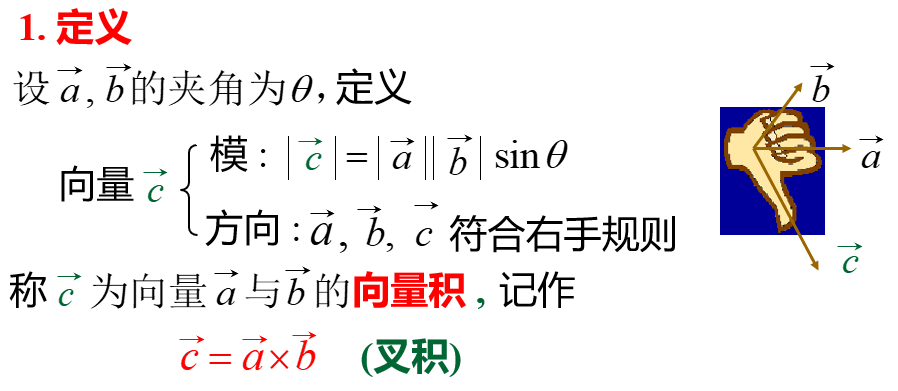这个是叉积结果与原来两个向量的方向与模长的关系，其中模长为两者模长相乘后乘以夹角的sin值，方向符合右手规则，即四指从第一个向量的方向以最小的角度转向第二个向量时的大拇指方向，这里区别第一个向量和第二个向量是因为叉积的结果与向量的顺序有关，于是有以下性质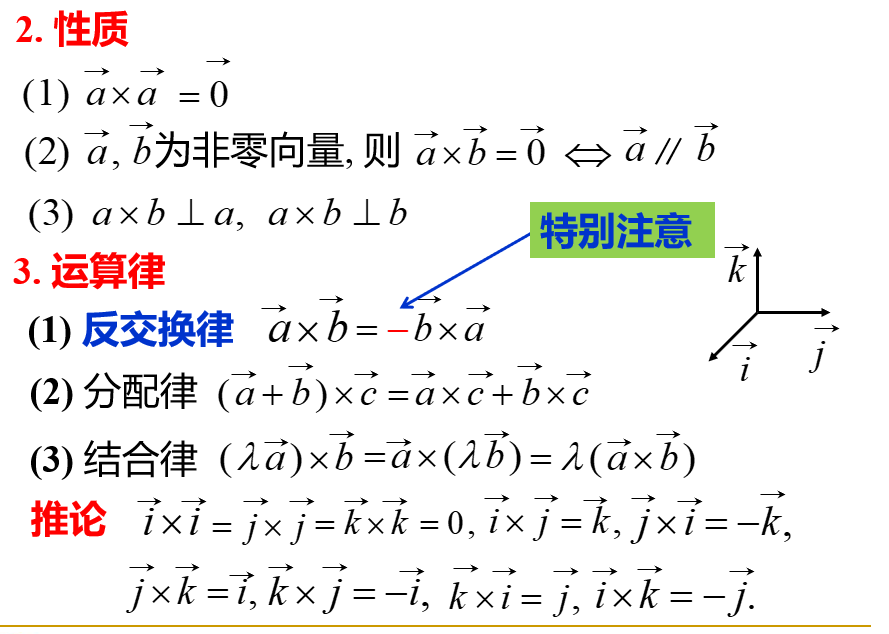然后是如果我们给定两个向量的坐标且已知个坐标的叉积结果，那么怎么求叉积结果的坐标，这里以三维空间为例：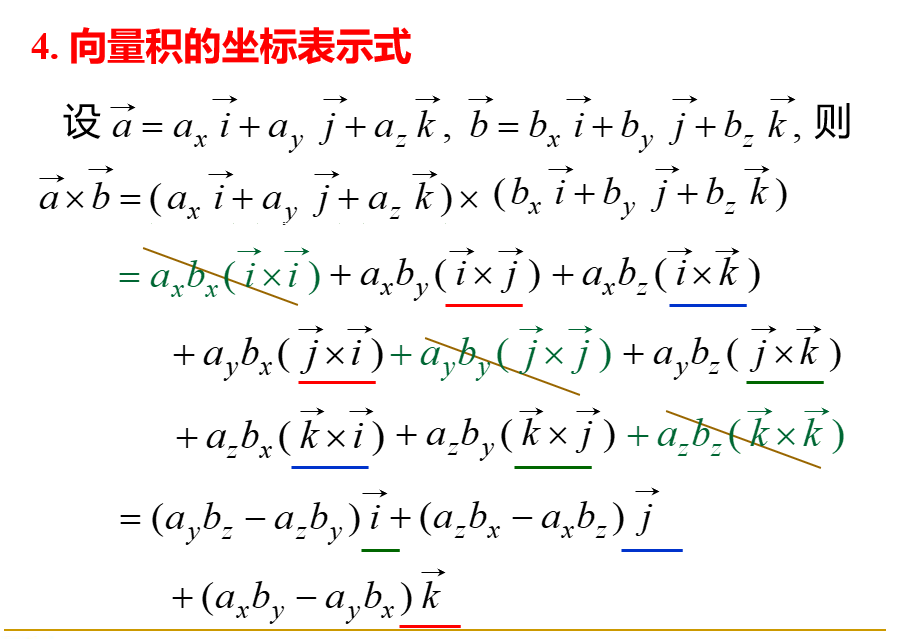结合前面的性质，我们很容易能将两个向量相乘的结果转化为坐标向量的叉积，那么对于正交的三维坐标系（两个坐标叉积结果与另一个共线），我们有一个有用的结论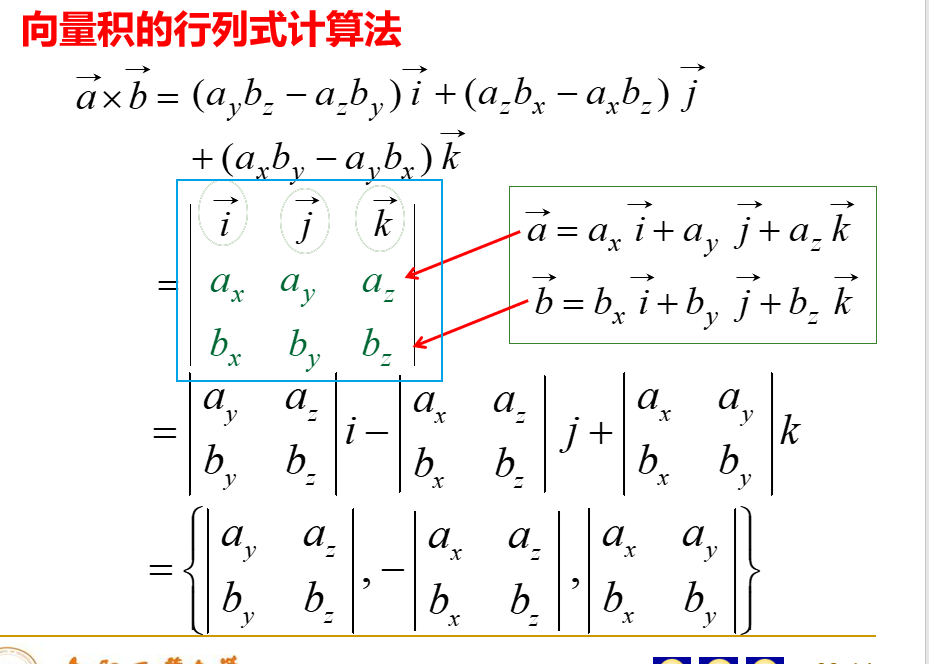有用的东西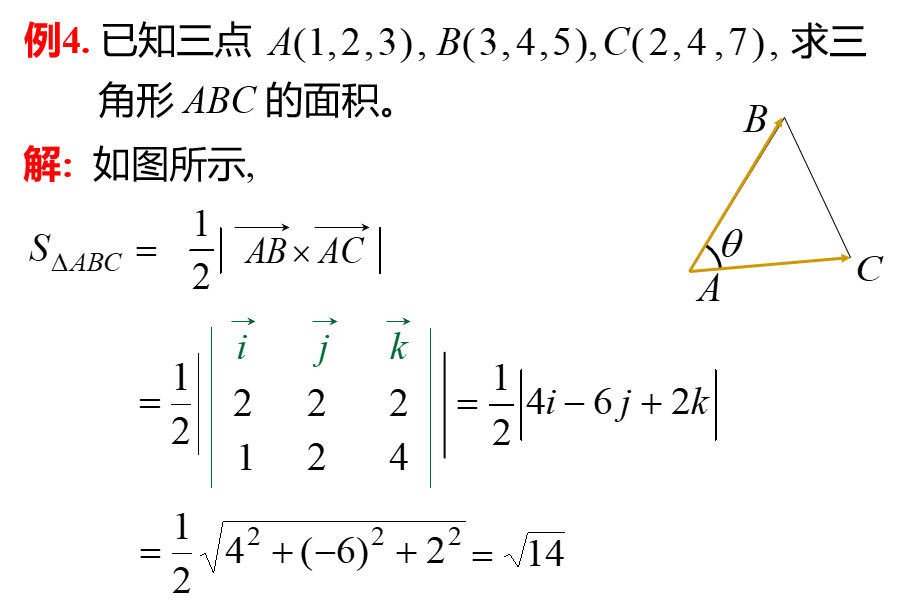叉积的结果刚好是两个向量三角区域的面积的两倍，这个可以帮助我们计算已知坐标的三角形面积的计算
混合积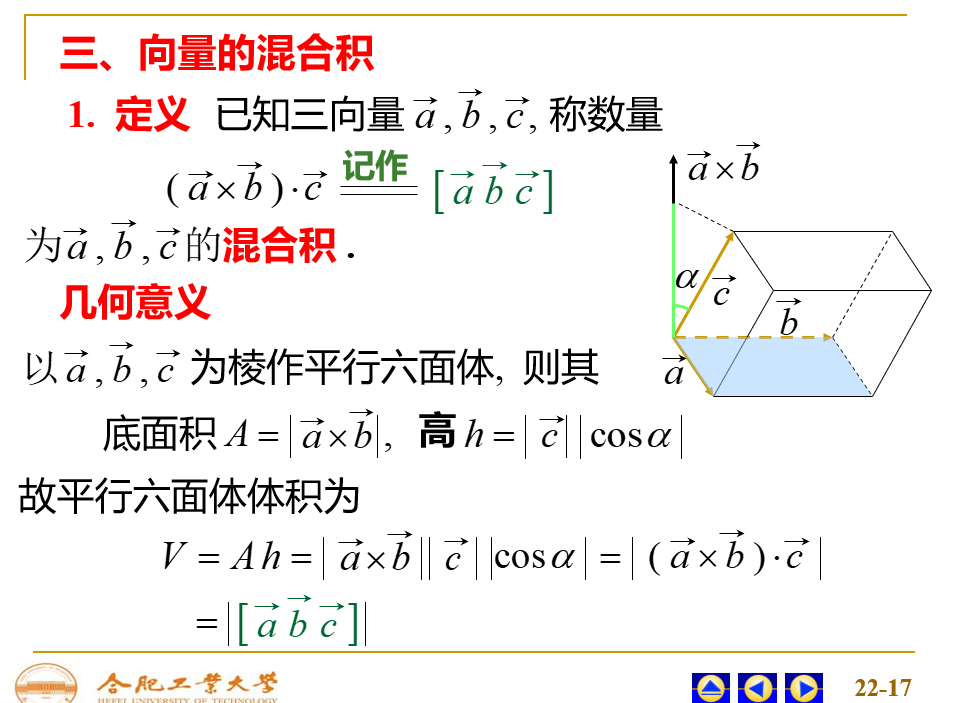由几何意义得轮换（不是乱换）值不变 坐标计算方法为：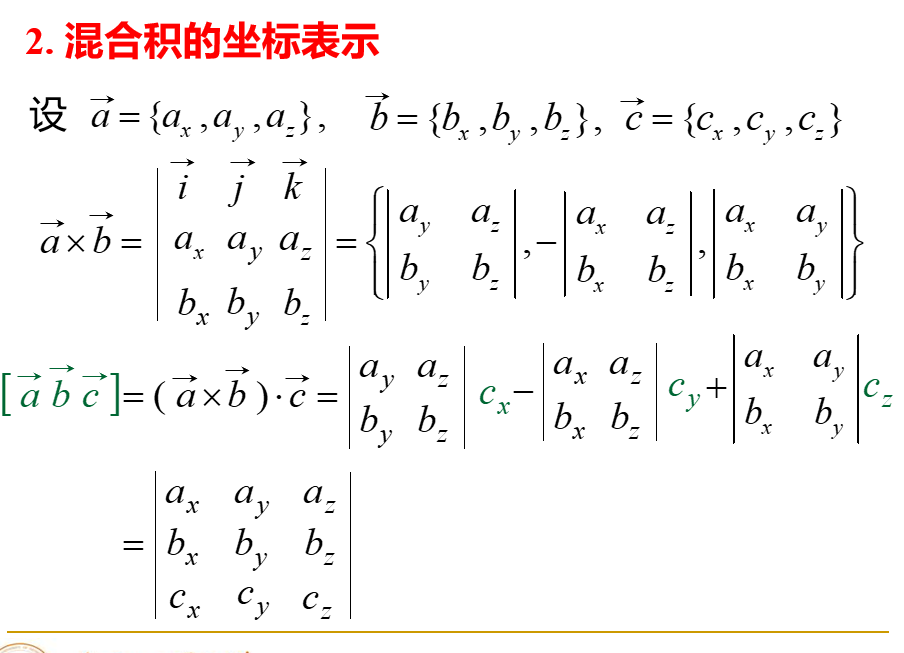由于ijk是单位向量相当于结果就是将ijk换成c的坐标，当然和这里给出的值是一样的，这里这样写我觉得就是方便记忆
有用的东西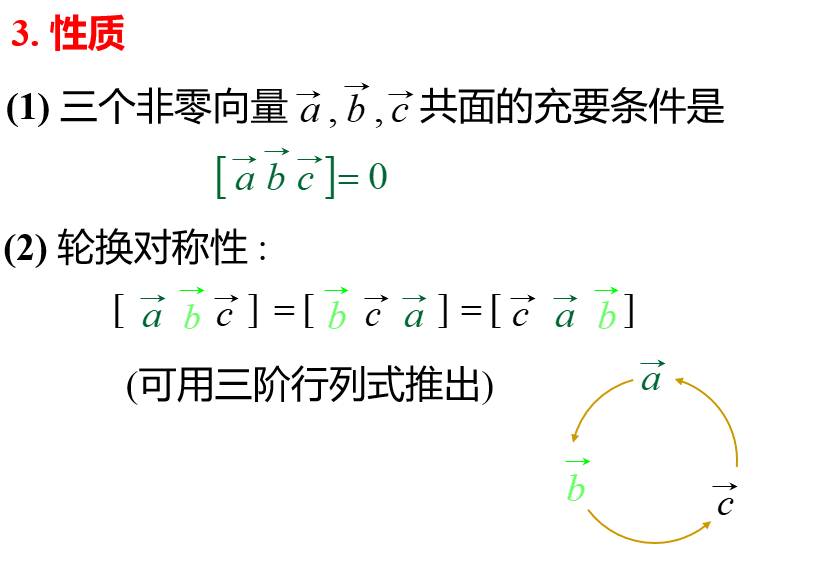平面
点法式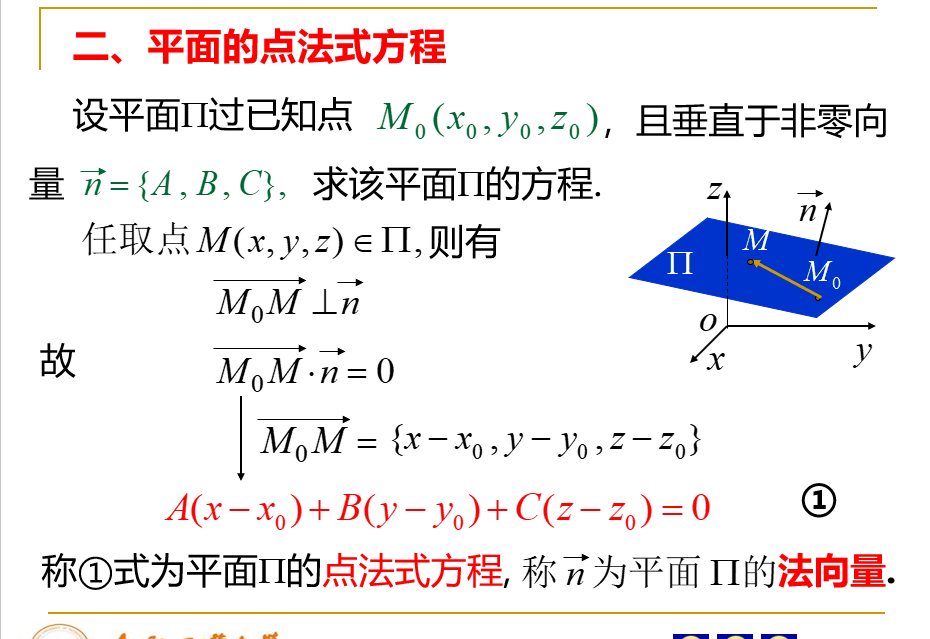关键是求出平面上的所有点满足的方程，现在知道一个点还有法向量，那么在平面上取一个点用它和那个点组成向量，这个向量与法向量的点积为零，就求完了
三点式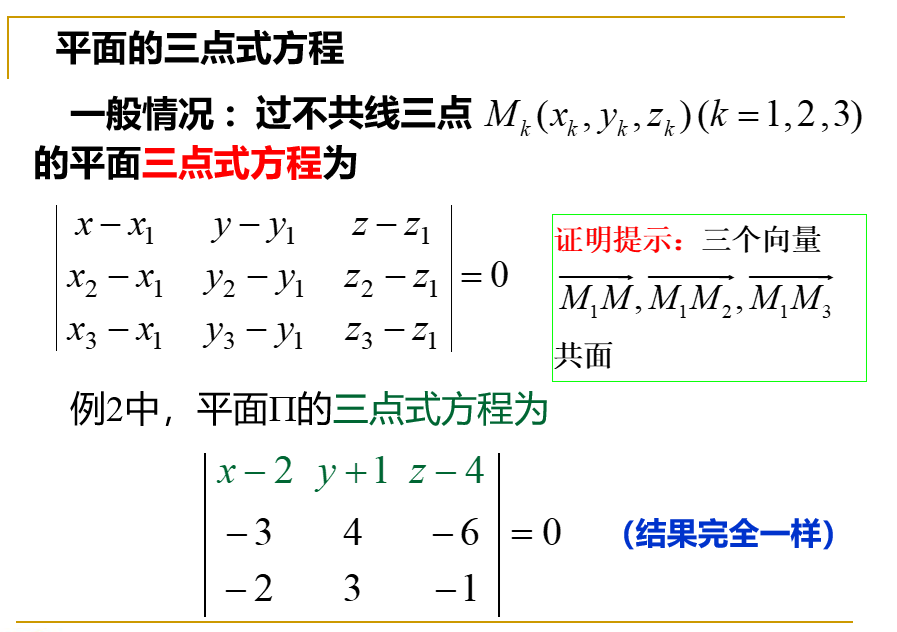共面就是三个向量的混合积为零，然后以减少x，y，z出现为标准选出三个向量就行了
截距式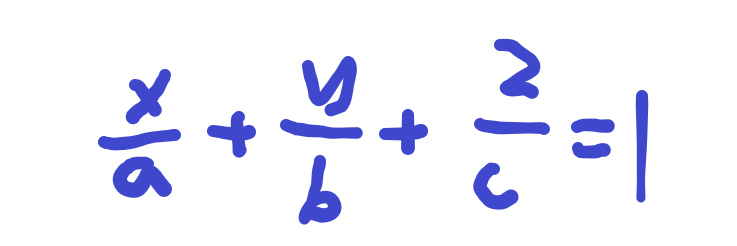平行与哪个轴，哪个轴对应的下面的数字就是无穷大，就是不存在这个数。
直线
一般式
就是两个平面方程联立
点向式（对称式）
要写方程，关键是找到直线上的满足的方程，已知一个点和方向向量，那么直线上的点与已知点的向量与方向向量平行，得到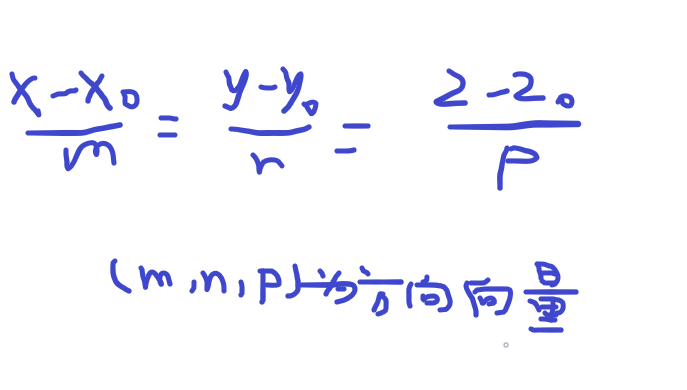参数式
可以由对称式快速转化过来。 令三个=t，将x，y，z用t表示
转化
关键是对称式和一般式的相互转换 一般式到对称式：两个平面叉积求出方向向量，然后再找一个点就行了。 对称式到一般式：就是两个等号连接的三个式子，随便取两个组合成两组作为方程联立后就是一般式方程。
补充
直线系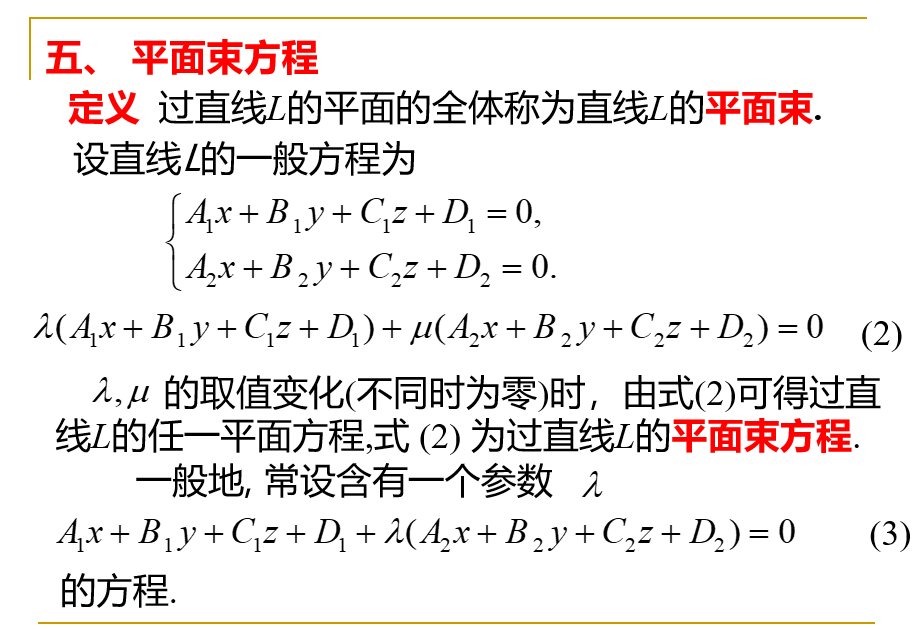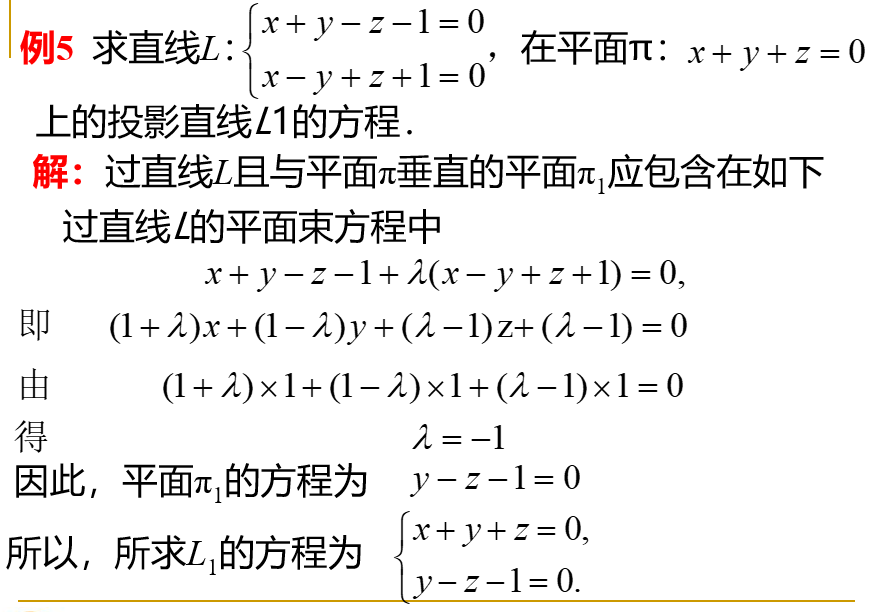类比二维中的直线系方程
点到平面的距离
思路是在平面上找一个点然后与外面那个点连成一个向量，这个向量在平面法向量上的投影就是点到平面的距离。记公式更好形式与二维中点到直线的距离公式形式差不多。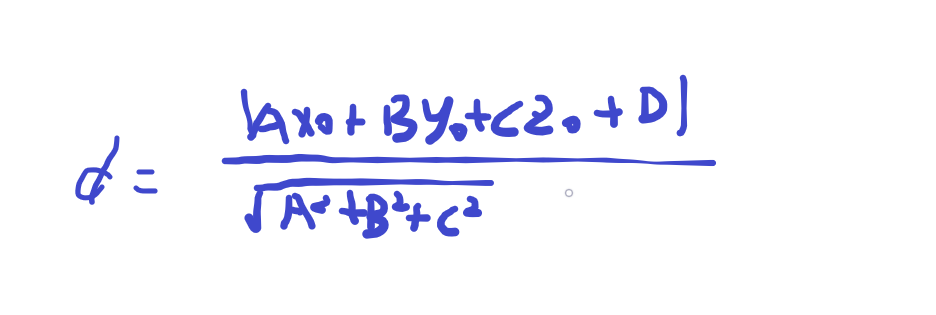判断两直线是否是异面的，顺便求距离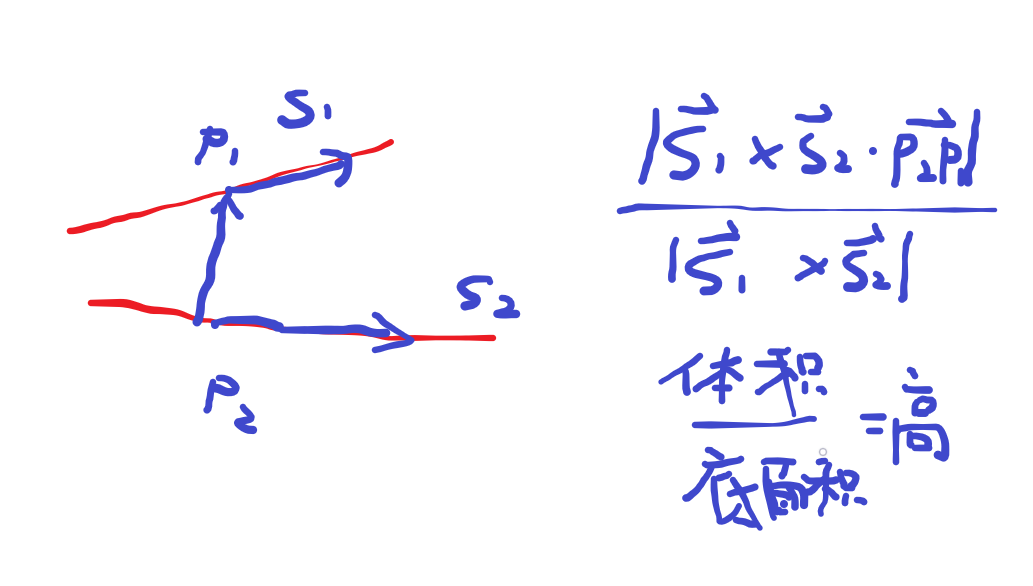就是这样，如果高是零就说明是共面的
已知直线与直线外一点求过该点且与直线垂直相交的直线
在直线上设一点（用参数式这样自带两个方程）（相交条件限定），再利用直线外那点与设的点的向量与直线方向向量垂直解出那点的坐标，进而得到方向向量，得到直线方程。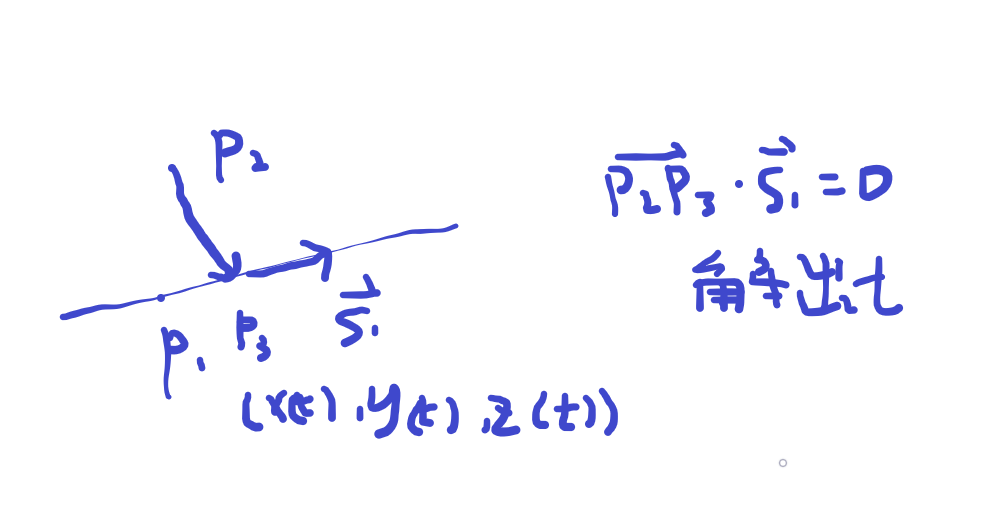利用s1和p2得到直线的垂面，再利用P1P2和s1的叉积得到方向向量，再结合P1或P2点写出平面方程，这两个方程联立就是所求直线的方程。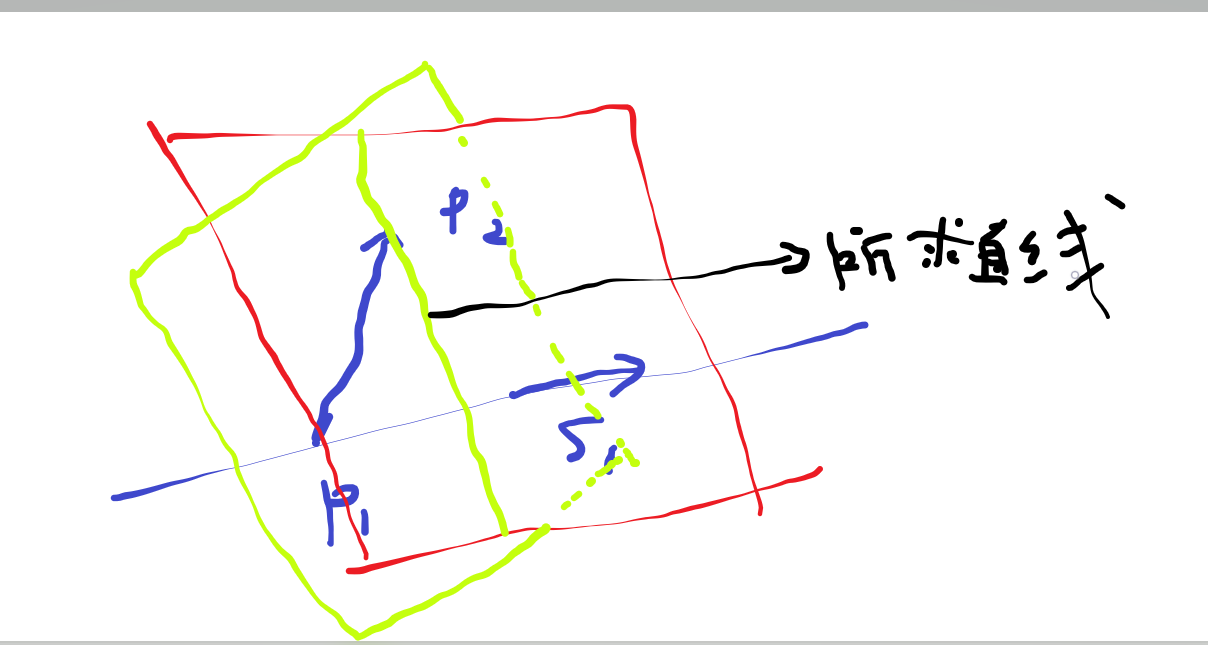3. 已经有了一个点了，还要方向向量，利用方向向量与s1垂直和方向向量与P1P2和s1的叉积垂直得到方向向量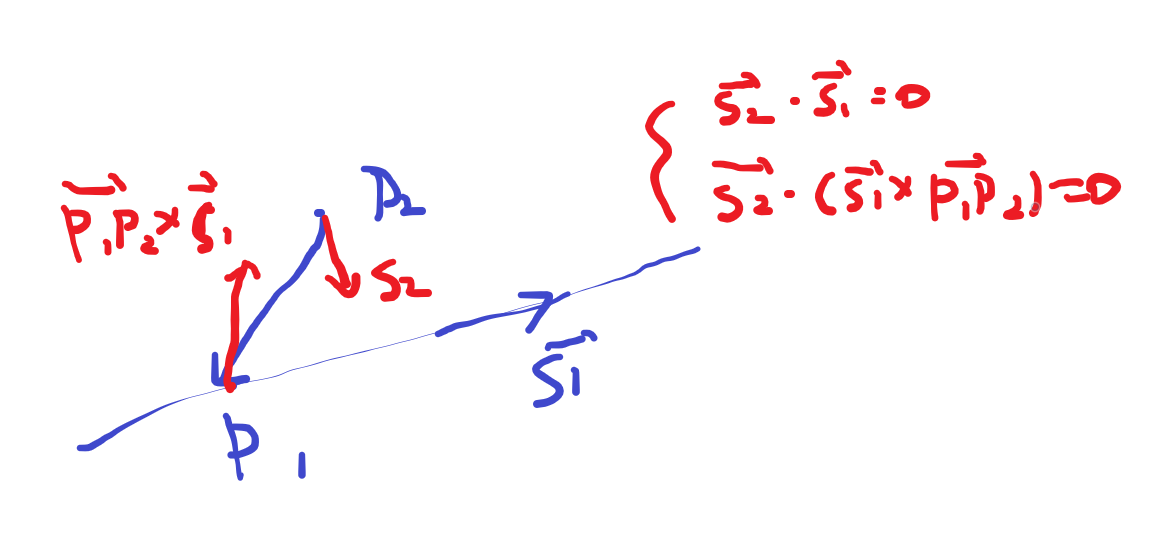异面直线的公垂线方程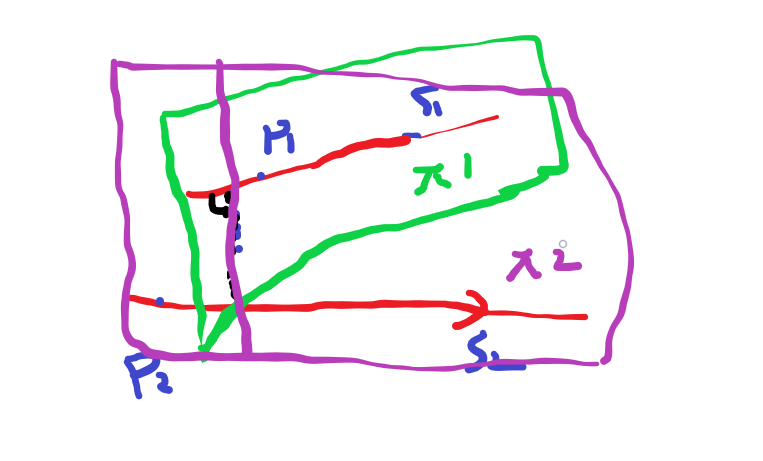方向向量很好求但是点很难找，所以我们采用平面联立的方式，两个平面已经在图中画出来了，他们分别过P1，P2点并且以方向向量与s1，s2的叉积为法向量。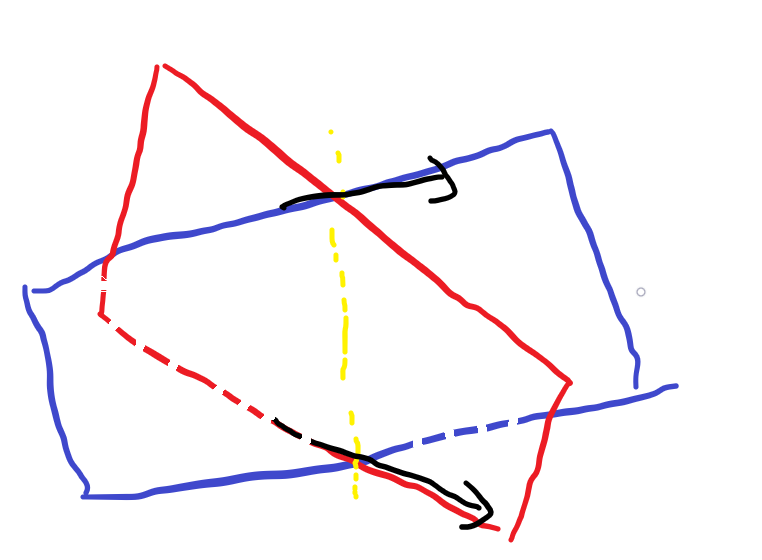求出红蓝平面的方程，他们的交线即为所求
展开全文• 1. 共线向量定理 对空间中任意两个向量，a⃗、b⃗（b⃗≠0），a⃗∥b⃗是存在唯一的实数 λ，使 a⃗=λb⃗ 2. 共面向量定理 如果两个向量 a⃗,b⃗ 不共线，则向量 p⃗ 与...3. 直线的向量参数方程 OP→=αOA...
• ## 参数方程

千次阅读 2010-07-05 13:33:00
在给定的平面直角坐标系中，如果曲线上任意一点的坐标x，y都是某个变数t的函数x=f(t),y=φ(t)，(1)且对于t的每一个允许值，由方程组(1)所确定的点m(x，y)都在这条曲线上，那么方程组(1)称为这条曲线的参数方程...
•人工智能 机器学习 数学
• 平面向量是在二维平面内既有方向(direction)又有大小(magnitude)的量，物理学中也称作矢量，与之相对的是只有大小、没有方向的数量（标量）。iOS动画 iOS绘图
• 它的参数方程为：其中，与分别对应单位向量与，它们既垂直于，又互相垂直；随着从0变化到，通过参数方程可以得到圆上每一个点的坐标。与是满足既垂直于，又互相垂直的任意单位向量。怎么样快速得到满足条件的与呢？...matlab画三维圆
• 平面方程　空间内不在同一直线上的三点构成一个平面平面方程可表示为ax + by + cz = d。平面方程也称为三元线性方程。　方程x + 4y + z = 8，在xyz三个坐标轴上的截距分别是(8,0,0),(0,2,0),(0,0...直线
• 平面及其方程问题的引入平面的点法式方程例1例2平面的截距方程特别的三点分别在三个坐标轴上平面的一般方程特殊情形的性质例3例4平面参数方程平面片的描绘点到平面的距离例6 平面截距与一点到平面的距离D的关系 ...算法
• ## 平面方程

千次阅读 2010-11-01 22:36:00
點法式： 若平面E法向量n=(a,b,c)且過點A(x0,y0,z0)，則平面E的方程式為a(x-x0)+b(y-y0)+c(z-z0)=0。 一般式： 將方程式a(x-x0)+b(y-y0)+c(z-z0)=0 化簡可得ax+by+cz+d=0的方程式。 我們...c
• ## 空间平面及其方程

千次阅读 2020-07-06 11:02:55
（A，B，C）为平面的法向量 三点式 一般方程 Ax + By + Cz + D = 0； （A，B，C）为平面的法向量 缺少谁，该平面就与哪个轴平行。 2. 常见问题 Π1：A1x + B1y + C1z + D1 = 0 Π2：A2x + B2y + C2z + D2 = ...
• 1. 问题的引入——通过网格精确描述和生成大飞机复杂外形 2. 平面的点法式方程（平面的法向量） ...3. 平面的三点式方程 ...6. 平面参数方程 7. 点到平面的距离公式 ...
• 这样就可以得到平面的两个向量p1p2(x2-x1,y2-y1,z2-z1),p1p3(x3-x1,y3-y1,z3-z1)，而平面法线总是和这两个向量垂直。也就是说，p1p2与p1p3的向量积就是平面的法向量n。 复习一下向量积，已知向量 a=(a1,a2,a3) b...
• 曲线 Geom_Circle Geom_Circle的参数方程OCCT 曲线 解析
• 设任意两个有序点P、Q对应于n维矢量空间中的一个矢量a，那么过两点的直线方程为：（1-t)P + tQ笛卡尔平面是一个仿射空间，那么空间两点（a,b), (c, d)之间的参数方程为：L = ｛((1-t)a + tc, (1-t)b + td) | t是...图形 3d
• 内容 参数化表达式 求平面向量 求点到平面的距离 求直线与平面交点 求三个平面斜交 1. 参数化表达式 ...除此之外，平面方程还有一种常用的表达方式： 这里的 ，，其实就是法向量的，，分量，而 2...计算机图形学
• B1，C1）是（1）的法向量，b→\overrightarrow {b}b=（A2，B2，C2）是（2）的法向量，记这两个平面的交线为l，则l必垂直于a和b构成的平面，而a+λb可用来表示所有过l的平面的法向量，这时候对应的平面方程就是我们的...